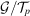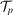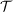International
Tables for
Crystallography
Volume A1
Symmetry relations between space groups
Edited by Hans Wondratschek and Ulrich Müller

International Tables for Crystallography (2006). Vol. A1, ch. 1.4, p. 27   | 1 | 2 |

## Section 1.4.3. Computing maximal subgroups

Franz Gählera*

aInstitut für Theoretische und Angewandte Physik, Universität Stuttgart, D-70550 Stuttgart, Germany
Correspondence e-mail: gaehler@itap.physik.uni-stuttgart.de

### 1.4.3. Computing maximal subgroups

| top | pdf |

The Cryst package has built-in facilities for computing the maximal subgroups of a given index for any space group. More precisely, given a prime number p, Cryst can compute conjugacy-class representatives of those maximal subgroups ofwhose index inis a power of p. The algorithms used for this task are described in Eick et al. (1997). Essentially, one determines the maximal subgroups of the (finite) factor group, whereis the subgroup of those translations ofwhich are a p-fold multiple of an element of the full translation groupof. After the maximal subgroups are obtained, the translations inare added back to the subgroups.

From a representativeof a conjugacy class of subgroups, the list of all subgroups in the same conjugacy class is obtained by repeatedly conjugating(and the subgroups obtained from it by conjugation) with generators of, until no new conjugated subgroups are obtained. This is also the way of determining whether two subgroups are conjugated: one enumerates the groups in the conjugacy class of one of them, and checks whether the other is among them.

The index of a subgroupofis easily computed as the product of the index of the point group ofin the point group ofand the index of the translation group ofin the translation group of. For maximal subgroups, only one of these factors is different from 1. For klassengleiche subgroups, the two point groups are equal, whereas for translationengleiche subgroups the two translation subgroups are equal. Therefore, a maximal subgroup is easily identified either as a klassengleiche or a translationengleiche subgroup.

### References

Eick, B., Gähler, F. & Nickel, W. (1997). Computing maximal sub-groups and Wyckoff positions of space groups. Acta Cryst. A53, 467–474.